# 图像处理 空间卷积原理

## 图像卷积-空间卷积

• ###### MATLAB图像处理

全面系统的学习MATLAB在图像处理中的应用

56课时 487分钟 21228人学习 魏伟
免费试看

### 空间卷积-Spatial convolutionApplet参见Applet链接

### 卷积示意：``````# #!/usr/bin/python
# # coding:utf-8

import cv2
import numpy as np

# # 卷积核
rect = np.array([[0, 0, 0, 0, 0],
[0, 0.11, 0.11, 0.11, 0],
[0, 0.11, 0.11, 0.11, 0],
[0, 0.11, 0.11, 0.11, 0],
[0, 0, 0, 0, 0]], np.float32)

bigrect = np.array([[0.04, 0.04, 0.04, 0.04, 0.04],
[0.04, 0.04, 0.04, 0.04, 0.04],
[0.04, 0.04, 0.04, 0.04, 0.04],
[0.04, 0.04, 0.04, 0.04, 0.04],
[0.04, 0.04, 0.04, 0.04, 0.04]], np.float32)

gaussian = np.array([[0.01, 0.02, 0.03, 0.02, 0.01],
[0.02, 0.06, 0.08, 0.06, 0.02],
[0.03, 0.08, 0.11, 0.08, 0.03],
[0.02, 0.06, 0.08, 0.06, 0.02],
[0.01, 0.02, 0.03, 0.02, 0.01]], np.float32)

sharpen = np.array([[0, 0, 0, 0, 0],
[0.02, 0.06, 0.08, 0.06, 0.02],
[0.03, 0.08, 0.11, 0.08, 0.03],
[0.02, 0.06, 0.08, 0.06, 0.02],
[0.01, 0.02, 0.03, 0.02, 0.01]], np.float32)

edges = np.array([[0, 0, 0, 0, 0],
[0, 0, -2, 0, 0],
[0, -2, 8, -2, 0],
[0, 0, -2, 0, 0],
[0, 0, 0, 0, 0]], np.float32)

shift = np.array([[0, 0, 0, 0, 0],
[0, 0, 0, 0, 0],
[1, 0, 0, 0, 0],
[0, 0, 0, 0, 0],
[0, 0, 0, 0, 0]], np.float32)

handshake = np.array([[0.2, 0, 0, 0, 0],
[0, 0.2, 0, 0, 0],
[0, 0, 0.2, 0, 0],
[0, 0, 0, 0.2, 0],
[0, 0, 0, 0, 0.2]], np.float32)

# 该函数实际上计算相关性，而不是卷积.  ddepth:目标图像的所需深度
rectimage = cv2.filter2D(img, -1, rect)
bigrectimage = cv2.filter2D(img, -1, bigrect)
gaussianimage = cv2.filter2D(img, -1, gaussian)
sharpenimage = cv2.filter2D(img, -1, sharpen)
edgesimage = cv2.filter2D(img, -1, edges)
shiftimage = cv2.filter2D(img, -1, shift)
handshakeimage = cv2.filter2D(img, -1, handshake)

cv2.imshow('original', img)
cv2.imshow('rect', rectimage)
cv2.imshow('bigrect', bigrectimage)
cv2.imshow('gaussian', gaussianimage)
cv2.imshow('sharpen', sharpenimage)
cv2.imshow('edges', edgesimage)
cv2.imshow('shift', shiftimage)
cv2.imshow('handshake', handshakeimage)

cv2.imwrite('map/rect.jpg',rectimage)
cv2.imwrite('map/bigrect.jpg',bigrectimage)
cv2.imwrite('map/gaussian.jpg',gaussianimage)
cv2.imwrite('map/sharpen.jpg',sharpenimage)
cv2.imwrite('map/edges.jpg',edgesimage)
cv2.imwrite('map/shift.jpg',shiftimage)
cv2.imwrite('map/handshake.jpg',handshakeimage)

cv2.waitKey(0)``````## 数字图像处理中相关和卷积的区别

2015-07-09 21:39:56 u012786955 阅读数 4590
• ###### MATLAB图像处理

全面系统的学习MATLAB在图像处理中的应用

56课时 487分钟 21228人学习 魏伟
免费试看

（1）移动相关核的中心元素，使它位于输入图像待处理像素的正上方
（2）将输入图像的像素值作为权重，乘以相关核
（3）将上面各步得到的结果相加做为输出

（1）卷积核绕自己的核心元素顺时针旋转180度
（2）移动卷积核的中心元素，使它位于输入图像待处理像素的正上方
（3）在旋转后的卷积核中，将输入图像的像素值作为权重相乘
（4）第三步各结果的和做为该输入像素对应的输出像素

可以看出他们的主要区别在于计算卷积的时候，卷积核要先做旋转。

f 函数
w 滤波器模板
eg：
f（x，y）
0 0 0 0 0
0 0 0 0 0
0 0 1 0 0
0 0 0 0 0
0 0 0 0 0
w（x，y）
1 2 3
4 5 6
7 8 9

0     0     0     0     0
0     9     8     7     0
0     6     5     4     0
0     3     2     1     0
0     0     0     0     0

0     0     0     0     0
0     1     2     3     0
0     4     5     6     0
0     7     8     9     0
0     0     0     0     0

0 0 0 0 0
0 1 2 3 0
0 4 5 6 0
0 7 8 9 0
0 0 0 0 0
f*w是多少呢？
9    26    50    38    21
42    94   154   106    54
90   186   285   186    90
54   106   154    94    42
21    38    50    26     9

1^2+2^2+……+9^2 = 285

## 图像卷积原理及运算方法

2018-06-07 15:39:46 u013539952 阅读数 38055
• ###### MATLAB图像处理

全面系统的学习MATLAB在图像处理中的应用

56课时 487分钟 21228人学习 魏伟
免费试看

## 运算方法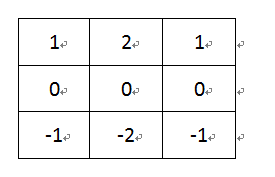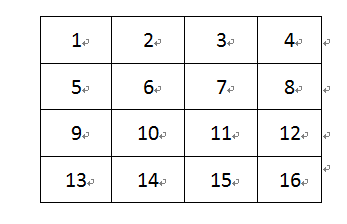1. 将卷积核旋转180°，即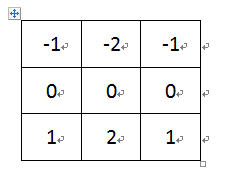2. 将卷积核h的中心对准x的第一个元素，然后h和x重叠的元素相乘，h中不与x重叠的地方x用0代替，再将相乘后h对应的元素相加，得到结果矩阵中Y的第一个元素。如：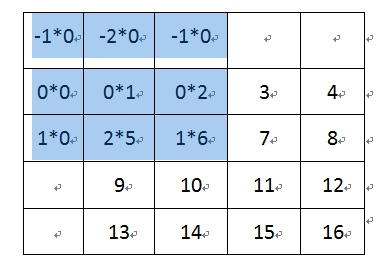所以结果矩阵中的第一个元素Y11 = -1 * 0 + -2 * 0 + -1 * 0 + 0 * 0 + 0 * 1 + 0 * 2 + 1 * 0 + 2 * 5 + 1 * 6 = 16

3. x中的每一个元素都用这样的方法来计算，得到的卷积结果矩阵为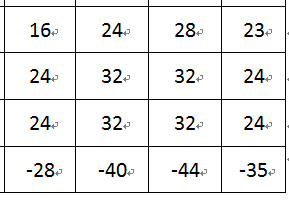## 图像处理——卷积原理、二维卷积python实现

2017-12-12 01:19:00 weixin_34160277 阅读数 278
• ###### MATLAB图像处理

全面系统的学习MATLAB在图像处理中的应用

56课时 487分钟 21228人学习 魏伟
免费试看

f(x) =  - 其中C(k)代表卷积操作数，g(i)代表样本数据, f(x)代表输出结果。1.      读取源图像像素

2.      应用卷积操作数矩阵产生目标图像

3.      对目标图像进行归一化处理

4.      处理边界像素

模板：矩阵方块，其数学含义是一种卷积运算。
卷积运算：可看作是加权求和的过程，使用到的图像区域中的每个像素分别于卷积核(权矩阵)的每个元素对应相
乘，所有乘积之和作为区域中心像素的新值。
卷积核：卷积时使用到的权用一个矩阵表示，该矩阵与使用的图像区域大小相同，其行、列都是奇数，
是一个权矩阵。
卷积示例：
3 * 3 的像素区域R与卷积核G的卷积运算：
R5(中心像素)=R1G1 + R2G2 + R3G3 + R4G4 + R5G5 + R6G6 + R7G7 + R8G8 + R9G9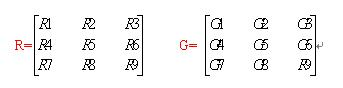边界问题：当处理图像边界像素时，卷积核与图像使用区域不能匹配，卷积核的中心与边界像素点对应，
卷积运算将出现问题。
处理办法：
A． 忽略边界像素，即处理后的图像将丢掉这些像素。
B． 保留原边界像素，即copy边界像素到处理后的图像。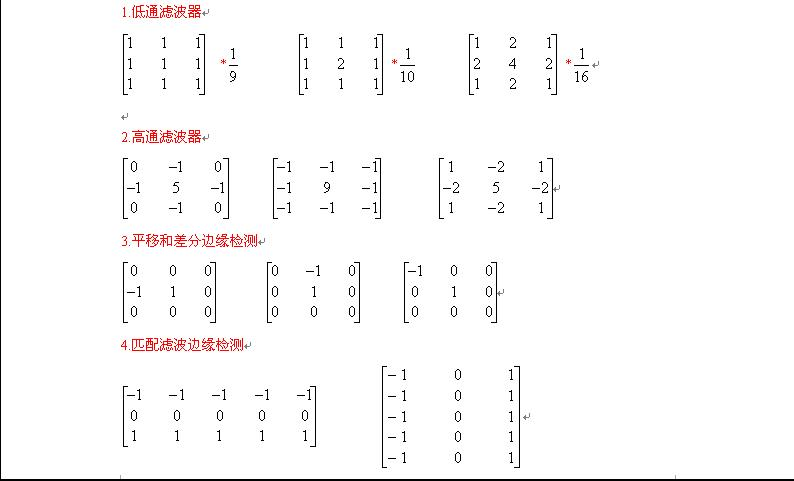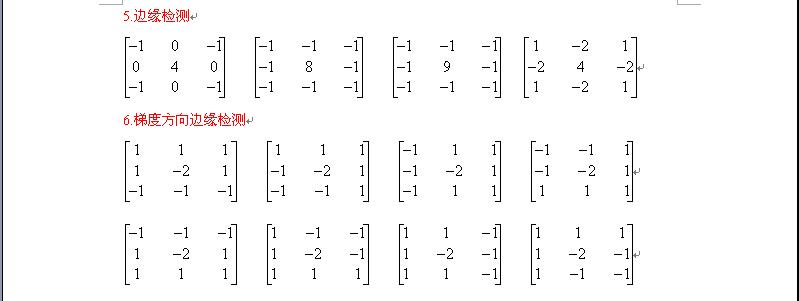-----------------------------------------------------------------------------------------------------------

以下用\$符号表示从负无穷大到正无穷大的积分。

一维卷积：
y(t)=g(k)*x(k)=\$g(k)x(t-k)
先把函数x(k)相对于原点反折，然后向右移动距离t，然后两个函数相乘再积分，就得到了在t处的输出。对每个t值重复上述过程，就得到了输出曲线。

二维卷积：
h(x,y)=f(u,v)*g(u,v)=\$\$f(u,v)g(x-u,y-v)
先将g(u,v)绕其原点旋转180度，然后平移其原点，u轴上像上平移x，   v轴上像上平移y。然后两个函数相乘积分，得到一个点处的输出。

```import numpy as np
from scipy import signal
from scipy import misc
import matplotlib.pyplot as plt
face=misc.face(gray=True) #创建一个灰度图像
scharr=np.array([[-3-3j,0-10j,+3-3j],
[-10+0j,0+0j,+10+0j],
[-3+3j,0+10j,+3+3j]]) #设置一个特殊的卷积和
fig,(ax1,ax2)=plt.subplots(1,2,figsize=(10,6)) #建立1行2列的图fig
ax1.imshow(face,cmap='gray') #显示原始的图
<matplotlib.image.AxesImage object at 0x00000000078FC198>
ax1.set_axis_off() #不显示坐标轴
<matplotlib.image.AxesImage object at 0x00000000078FCE48>
ax2.set_axis_off() #不显示坐标轴
fig.show() #显示绘制好的画布```

## 彻底理解数字图像处理中的卷积-以Sobel算子为例

2017-11-07 18:13:51 qq283980619 阅读数 6343
• ###### MATLAB图像处理

全面系统的学习MATLAB在图像处理中的应用

56课时 487分钟 21228人学习 魏伟
免费试看

## 概述## 以 Sobel 算子为例

Sobel 算子 也叫 Sobel 滤波, 是两个 3*3 的矩阵, 主要用来计算图像中某一点在横向/纵向上的梯度, 看了不少网络上讲解 Sobel 算子 的文章, 发现人们常常把它的横向梯度矩阵和纵向梯度矩阵混淆. 这可能与 Sobel 算子 在它的两个主要应用场景中的不同用法有关.

Sobel 算子的两个梯度矩阵: Gx 和 GyO ——-> x轴
|
|
|
V y轴

Sobel 算子的用途

• 边缘检测时: Gx 用于检测纵向边缘, Gy 用于检测横向边缘.
• 计算法线时: Gx 用于计算法线的横向偏移, Gy用于计算法线的纵向偏移.

Gy 计算展开为:

a b c
d e f
g h i

i f c
h e b
g d a

i h g
f e d
c b a

convolution is the process of flipping both the rows and columns of the kernel and then multiplying locationally similar entries and summing.

g h i
d e f
a b c

i h g
f e d
c b a## 图像边缘像素的处理

``````// 用 sobel 算子生成法线图 generate normal map with sobel operator

genNormal1 = {
attribute vec4 position;
attribute vec4 color;
attribute vec2 texCoord;

varying vec2 vTexCoord;
varying vec4 vColor;
varying vec4 vPosition;

uniform mat4 modelViewProjection;

void main()
{
vColor = color;
vTexCoord = texCoord;
vPosition = position;
gl_Position = modelViewProjection * position;
}
]],

precision highp float;

varying vec2 vTexCoord;
varying vec4 vColor;
varying vec4 vPosition;

// 纹理贴图
uniform sampler2D tex;
uniform sampler2D texture;

//图像横向长度-宽度, 图像纵向长度-高度
uniform float w;
uniform float h;

float clamp1(float, float);
float intensity(vec4);

float clamp1(float pX, float pMax) {
if (pX > pMax)
return pMax;
else if (pX < 0.0)
return 0.0;
else
return pX;
}

float intensity(vec4 col) {
// 计算像素点的灰度值
return 0.3*col.x + 0.59*col.y + 0.11*col.z;
}

void main() {
// 横向步长-每像素点宽度，纵向步长-每像素点高度
float ws = 1.0/w ;
float hs = 1.0/h ;
float c;
vec2 p = vTexCoord;
lowp vec4 col = texture2D( texture, p );

// sobel operator
// position.      Gx.            Gy
// 1 2 3     |-1. 0. 1.|   |-1. -2. -1.|
// 4 5 6     |-2. 0. 2.|   | 0.  0.  0.|
// 7 8 9     |-1. 0. 1.|   | 1.  2.  1.|
// 右上角，右，右下角

c = intensity(texture2D( texture, vec2(clamp(p.x+ws,0.,w), clamp(p.y+hs,0.,h) )));
c = intensity(texture2D( texture, vec2(clamp1(p.x+ws,w), clamp1(p.y,h))));
c = intensity(texture2D( texture, vec2(clamp1(p.x+ws,w), clamp1(p.y-hs,h))));

// 上, 下
c = intensity(texture2D( texture, vec2(clamp1(p.x,w), clamp1(p.y+hs,h))));
c = intensity(texture2D( texture, vec2(clamp1(p.x,w), clamp1(p.y-hs,h))));

// 左上角, 左, 左下角
c = intensity(texture2D( texture, vec2(clamp1(p.x-ws,w), clamp1(p.y+hs,h))));
c = intensity(texture2D( texture, vec2(clamp1(p.x-ws,w), clamp1(p.y,h))));
c = intensity(texture2D( texture, vec2(clamp1(p.x-ws,w), clamp1(p.y-hs,h))));

// 先进行 sobel 滤波, 再把范围从 [-1,1] 调整到 [0,1]
// 注意: 比较方向要跟坐标轴方向一致, 横向从左到右, 纵向从下到上
float dx = (c+2.*c+c-(c+2.*c+c) + 1.0) / 2.0;
float dy = (c+2.*c+c-(c+2.*c+c) + 1.0) / 2.0;
float dz = (1.0 + 1.0) / 2.0;

gl_FragColor = vec4(vec3(dx,dy,dz), col.a);

}
]]
}``````